# FlightSimulator3

## FlightSimulator3

In this section, we make the final version of the flight simulator game (see Figure 5.20), in which we add a gauge-type compass (which will replace the impractical strings that we used previously to tell us the compass heading), a rate-of-climb indicator, and a roll indicator. In addition, we will adjust the roll and yaw controls so that the motion is more realistic, and we will add a few sounds to the game.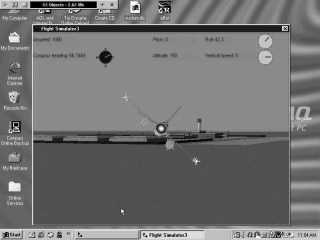Figure 5.20: The final flight simulator with analog type instruments. The compass heading in particular is useful in landing the jet properly.

The topics we will learn about include the following:

• Working with sprites

• Antialiasing and transparent colors

A sprite is a two-dimensional figure that you can display on your screen. You can make it move anywhere , change its angle, or just let it sit there. You ve probably seen sprites on web pages; they may have taken the form of something like a cartoon that moves across the display to get your attention.

It s important to realize that a sprite is not an object in your 3D game world. Think of the sprite as living on your screen, but one that never moves into the third dimension of the screen. The location of the sprite does not change as the camera moves around and remains on your screen unless you give it a specific command to move. These characteristics explain why sprites are a great way to display instruments on the screen. If sprites were objects in the 3D world, they would need to follow the camera in order to remain visible ”this is too complicated!

Each instrument is going to be made up of two separate images or sprites: a round gauge and a rod-like indicator that rotates on top of the gauge. The gauge is stationary, and the indicator rotates as speed or headings change. These items are shown in Figure 5.20; the compass gauge is in the upper left, composed of a round gauge and an indicator.

On the CD    The first thing we are going to do is make the stationary gauge used in the rate-of-climb and roll indicators using the Jamagic picture editor. (You do not need to make these sprites since they can be found on the CD-ROM, but it s great practice for you to try your hand at it. If you do import them from the CD-ROM, please see the following important Tip.) The gauge is a 100 x 100 image that consists of a circle filled in with a light gray color , called simply Gauge (see Figure 5.21). The entire sprite is made up of a rectangle with the filled-in circle inside the rectangle. It s important that you fill only the circle with color and not the rectangle around it; if you accidentally fill the rectangle, it will be visible on the screen. As long as the rectangle isn t mistakenly filled in, it will be invisible. This is because the white color of the rectangle is the transparent color for Jamagic ”anything that particular shade of white is ignored by the program. A transparent color is necessary in order to make the drawing of figures easier.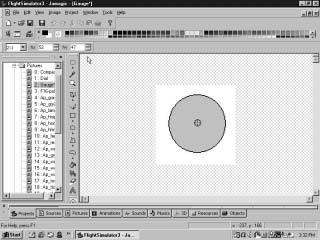Figure 5.21: The gauge background sprite. Note that the rectangular area is left as the original white color, which is transparent to Jamagic . Also note the hot-spot location at the center of the gauge and the hot-spot icon beneath the text icon.

Every picture in Jamagic has a hot spot. If you tell Jamagic to put the sprite at screen coordinates (120, 120), then the hot spot will be put at that location. Also, if you tell Jamagic to rotate the sprite, the sprite will rotate about the hot spot.

By default the hot spot is placed at the upper-left corner of the image. But in Figure 5.21, the hot spot has been moved to the center of the circle. You can recognize the hot spot because it appears as a small circle with an X at the center. By clicking the hot-spot icon located beneath the text icon, you can make the hot spot visible, and you can move it around with the mouse. If the hot-spot icon is not visible, you will have to drag the lower boundary of the picture editor down with your mouse, since the hot-spot icon is near the lower edge of the picture editor window.

 Tip If you import a sprite from another program or elsewhere, you have to remember that sometimes the hot spot shifts back to the upper-left corner when it finishes importing. This can cause problems if the sprite is supposed to rotate about its hot spot. If you notice some strange behavior from a sprite, particularly in rotation, check the location of its hot spot using the picture editor.

Now is probably a good time to start a new program called FlightSimulator3 and to experiment with making the sprite. Make sure that you move the hot spot to the center of the circle.

We need the indicator which rotates over the gauge, showing the speed or heading. This is done by making another picture with the Jamagic picture editor called dial (see Figure 5.22). It s important that the hot spot of the indicator is at the bottom of the dial because this is the point about which the indicator rotates.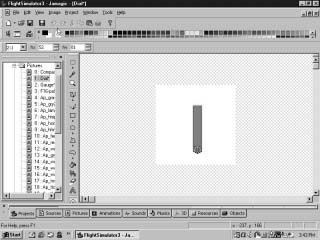Figure 5.22: The indicator dial. Note that the hot spot is at the bottom.

Next we make the gauge for the compass, again putting the hot spot at the center of the gauge. We also use the text tool to write in the directions (see Figure 5.23).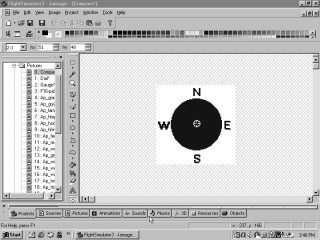Figure 5.23: The compass gauge with compass directions in bold letters .

Now that we have the graphics, we need the code to move the indicator over the gauge. Consider the following lines of code, which should be placed outside of the game loop and after the 3D world is created. These lines make a new sprite at location 580, 20 using the gauge picture we just made (Figure 5.23) scaled down by 0.5:

` // Roll gauge mygauge = New Sprite(580,20,"gauge"); mygauge.SetScale(0.5,0.5); `

How did we know to put the gauge at (580, 20) and to scale it by one half? This was determined by a trial and error process. We took into account that the game will be using a resolution of 640 x 480 pixels in order to estimate the location of the gauge at (580, 20). This resolution gives us a rough idea of where to place the gauge, and trial and error is used for fine-tuning the location.

Next we put the indicator in, also at (580, 20). In the following code, the last line is unnecessary. It is there merely to illustrate that the sprite can be moved using the Set command:

` //Roll pointer mysprite = New Sprite(580,20,"dial"); mysprite.SetScale(0.25,0.25); mysprite.Set(580,20); `

We can rotate the gauge with the following command, which must go inside of the game loop (note that the previous statements are placed before the loop):

` mysprite.SetAngle(roll2,FALSE); `

This command tells Jamagic to rotate the sprite roll2 degrees, which is the jet s angle of roll. It s interesting to note that this is just about the only function that takes degrees and not radians! The FALSE tells Jamagic not to use antialiasing with the sprite.

Any picture on a computer screen is made up of tiny rectangular dots called pixels . Unfortunately, these can sometimes look rough and jagged, something like a stairway seen from the side, so a technique known as antialiasing is used. Antialiasing makes the colors of the image edges blend in with the colors of the background, making the border between the two fuzzy. This makes the image look smoother because you do not see the jagged edges. If you look at most stationary larger images on your screen, you will be able to see this. Unfortunately antialiasing takes the computer more time to compute, so we disable it by putting FALSE in the parentheses in the code just listed. It makes little difference in this case.

We also wish to have a vertical speed (rate-of-climb) indicator. Typically, this consists of a gauge similar to the one we just made for roll, except that the indicator is horizontal. Ascents result in a counter-clockwise rotation (pitching up) of the indicator, while descents point the indicator down; this is easy for the pilot to understand with a quick glance. We can use the same gauge and indicator as before; we just need to rotate the indicator 90 degrees to make it horizontal:

` //Vertical speed gauge myvgauge = New Sprite(580,60,"gauge"); myvgauge.SetScale(0.5,0.5); //Vertical speed pointer myvsprite = New Sprite(580,60,"dial"); myvsprite.SetScale(0.25,0.25); myvsprite.SetAngle(90,FALSE); `

For the case of the roll angle, it was very easy to relate the roll angle to the angle on the indicator. Things are not so simple for the rate-of-climb, however. This is because we have to make the indicator turn further as the vertical speed increases . We can do this by setting a proportion. For example, we can say that one revolution of the indicator (360 degrees) will correspond to 100 miles per hour, so if the vertical speed is 50 miles per hour down, then the indicator should turn half a revolution (180 degrees) down. If the vertical speed is 25 miles per hour down, the indicator would turn one-quarter revolution down, and so forth ”the greater the speed, the greater the amount of revolution of the indicator.

We can make a mathematical expression to express this proportion between rotation and vertical speed, by saying 100 miles per hour is to 360 degrees as vertical speed is to indicator angle:

` (100) / (360) = (vertical speed) / (indicator degrees rotation). `

Solving the above for the degrees of rotation gives us

` indicator degrees of rotation = current vertical speed * 360/100. `

The code for setting the angle of rotation x of the indicator is then

` x = 1* vert*360/1000 + 90.; myvsprite.SetAngle(x,FALSE); `

The compass gauge uses a different sprite for the background since we are making it black, but it uses the same indicator as in the previous gauges:

` //Compass gauge mycgauge = New Sprite(180,60,"compass"); mycgauge.SetScale(0.5,0.5); //Compass pointer mycsprite = New Sprite(180,60,"dial"); mycsprite.SetScale(0.25,0.25); mycsprite.Set(180,60); `

We can easily set the compass sprite angle using the degs value calculated earlier, which is the heading of the jet in degrees:

` mycsprite.SetAngle(degs,FALSE); `

We could also make gauges for the airspeed and the pitch, but nothing new would be learned from the effort, and they do not make the game any easier to play. The vertical speed gauge and compass gauges, on the other hand, will be quite useful in helping you land the jet properly.

We will also add two sounds, which you must import into Sounds before you run your program. The first is a voice that will say, I like it! if a successful landing is made, while the other is an obnoxious laugh that will be played if an unsuccessful landing is made. These sounds can be found on the Jamagic full-capability CD-ROM, and on the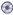FlightSimulator3 simulator program.

` victor = New Sound("Ilikeit"); loser = New Sound("Laugh08"); `

We now present the full code. Bear in mind that the digital instrument displays have been moved around, the turning rates have been changed from the previous game, and the text compass readings have been eliminated. In addition, sounds have been installed to congratulate the pilot for a proper landing, and to berate him for a poor one.

### FlightSimulator3 Code

` // Will add: // analog instruments using sprites, // "or" statement owindow1 = New Window("Flight Simulator3",640,480,Window.STANDARD);//one line //Window maintenance owindow1.SetAutoRefresh(OFF); // Sounds victor = New Sound("Ilikeit"); loser = New Sound("Laugh08"); // New world oworld = New World(); //Import jet. oworld.Load("f16"); myjet= oworld.GetObject(oworld.GetNObjects()1); myjet.Scale(0.01); myjet.SetPosition(0,150,2000); // Airport oworld.Load("airport"); mountain = oworld.GetObject(oworld.GetNObjects()1); mountain.Scale(0.05,0.05,0.05); mountain.SetPosition(0,0,4000); mountain.SetStatic(); // New cameras ocamera1 = New Camera(oworld,owindow1); ocamera1.SetBackgroundColor(GetRGB(0,150,200)); // Camera ocamera1.Follow(myjet,100); oworld.Optimize(ocamera1); //ocamera1.SetFocus(30); // Text boxes mytext = New StaticText(owindow1," ",0,10,110,20); mytext.SetBackColor(GetRGB(0,150,200)); mytext.SetColor(GetRGB(0,0,0)); mytext2 = New StaticText(owindow1," ",0,50,140,20); mytext2.SetBackColor(GetRGB(0,150,200)); mytext2.SetColor(GetRGB(0,0,0)); mytext3 = New StaticText(owindow1," ",300,50,110,20); mytext3.SetBackColor(GetRGB(0,150,200)); mytext3.SetColor(GetRGB(0,0,0)); mytext4 = New StaticText(owindow1," ",300,10,110,20); mytext4.SetBackColor(GetRGB(0,150,200)); mytext4.SetColor(GetRGB(0,0,0)); mytext5 = New StaticText(owindow1," ",430,10,70,20); mytext5.SetBackColor(GetRGB(0,150,200)); mytext5.SetColor(GetRGB(0,0,0)); mytext6 = New StaticText(owindow1," ",430,50,130,20); mytext6.SetBackColor(GetRGB(0,150,200)); mytext6.SetColor(GetRGB(0,0,0)); //Set collision detection. oworld.OnCollide = docollision; myjet.SetCollision(TRUE,Object.COLLISION_TYPE_STOP); // Initialize variables. airspeed = 0.0; alt = 0.0; thetax = 0.0; thetay = 0.0; thetaz = 0.0; degs = 0.0; speed = 0.0; addfactor = 0.0; leftfactor = 0.0; xpos = 0.; ypos = 150.; zpos = 0.0; Pitch2 = 0.0; roll2 = 0.0; rolldegs = 0.0; stopflag = FALSE; vert = 0.0; xco = 0.0; zco = 0.0; icount = 0; //Roll gauge mygauge = New Sprite(580,20,"gauge"); mygauge.SetScale(0.5,0.5); //Roll pointer mysprite = New Sprite(100,100,"dial"); mysprite.SetScale(0.25,0.25); mysprite.Set(580,20); //Vertical speed gauge myvgauge = New Sprite(580,60,"gauge"); myvgauge.SetScale(0.5,0.5); //Vertical speed pointer myvsprite = New Sprite(100,100,"dial"); myvsprite.SetScale(0.25,0.25); //The following one is in degrees, //the true refers to antialiasing myvsprite.SetAngle(90,FALSE); myvsprite.Set(580,60); //Compass gauge mycgauge = New Sprite(180,60,"compass"); mycgauge.SetScale(0.5,0.5); //Compass pointer mycsprite = New Sprite(180,60,"dial"); mycsprite.SetScale(0.25,0.25); //myvsprite.SetTransparent(ON); //The following one is in degrees, the true //refers to antialiasing mycsprite.Set(180,60); //Game loop While(1) { // Window cleaning     ocamera1.ActivateRender();     owindow1.Refresh(); //Set where to follow jet from. If(Keyboard.IsKeyDown(Keyboard.SHIFT))   { ocamera1.Follow(myjet,10,10,10,0);   } // Jet controls If(Keyboard.IsKeyDown(Keyboard.CAPSLOCK))   {     ocamera1.Follow(myjet,100);   } If(Keyboard.IsKeyDown(Keyboard.LEFT))  {    myjet.RollLeft(Pi/500.);    thetaz = thetaz  (Pi/500.);    If(thetaz< (2*Pi))      {      thetaz = 0.0;      }  } If(Keyboard.IsKeyDown(Keyboard.RIGHT))  {    myjet.RollRight(Pi/500.);    thetaz = thetaz + (Pi/500.);    If(thetaz>(2*Pi))      {      thetaz = 0.0;      }  } If(Keyboard.IsKeyDown(Keyboard.UP))  {    myjet.TurnUp(Pi/3000.);    thetax = thetax  (Pi/3000.);    If(thetax<(2*Pi))      {      thetax = 0.0;      }   } If(Keyboard.IsKeyDown(Keyboard.DOWN))  {    myjet.TurnDown(Pi/3000.);    thetax = thetax + (Pi/3000.);    If(thetax>(2*Pi))      {      thetax = 0.0;      }   } //Calculate airspeed based on pitch. airspeed = 1000  1000*Sin(thetax); // Move the aircraft as per the airspeed. speed = airspeed/100.; If(stopflag == FALSE) {   myjet.Move(speed); } //Instruments mytext.SetText("Airspeed: "+ airspeed); //Calculate compass heading in degrees. degs = 360.  thetay * 360.0/(2.*Pi) ; If (degs>360)  {    degs = degs  360;   } mytext2.SetText("Compass heading: " + degs); //Altitude alt = myjet.GetY(); mytext3.SetText("Altitude: " + alt); // Convert to degrees. pitch = thetax * 360./(2.*Pi); mytext4.SetText("Pitch: " + Pitch);  //Calculate vertical speed. vert = airspeed * Sin(Pitch *2*Pi/360); mytext6.SetText("Vertical Speed: " + vert); //Convert radians to degrees. rolldegs = thetaz * 360./(2.*Pi); //Send the roll angle to the screen. mytext5.SetText("Roll: " + rolldegs); //Move the jet to the side when jet rolls. // Right roll first If(thetaz >0.01)      {      leftfactor = 0.0004 * Cos(thetaz);      myjet.MoveRight(leftfactor);      addfactor = Pi/1500. * Cos(thetaz);    myjet.AddAngle(0,addfactor,0);      thetay = thetay+addfactor;      If(thetay>(2*Pi))         {         thetay = 0.0;         }      } // // Roll to left. If(thetaz < 0.01)      {      leftfactor = 0.0004 * Cos(thetaz);      myjet.MoveLeft(leftfactor);      addfactor = Pi/1500. * Cos(thetaz);      myjet.AddAngle(0,addfactor,0);      thetay = thetay+addfactor;      If(thetay < (2*Pi))       {        thetay=0.0;       }      } // xco = myjet.GetX; zco = myjet.GetZ; yco = myjet.GetY; //Analog instruments mysprite.SetAngle(roll2,FALSE); //Vertical speed need to relate degrees to speed //vert/100 = x/360 (each revolution of the pointer // will be 100 miles per hour) x = 1* vert*360/1000 + 90.; myvsprite.SetAngle(x,FALSE);  //Compass mycsprite.SetAngle(degs,FALSE); // End of game loop } Function docollision { // In order for landing to be successful, // several criteria must be met: // Heading must be between 268 and 272 // (must come in from the east). // Vertical speed vert must be less than 30 miles per //hour. // Landing must be between x = 2310 and 1770. // z must be between 6063 and 5241 //(location of landing area).       If(degs >= 268 && degs <=272)        {         If(vert>30 && zco < 5241 && zco >6063&&                 xco <1770 &&//one line       xco> 2310)//one line         {         SetText("Landed correctly!");          victor.Play();         myjet.Stop();         stopflag = TRUE;         Return stopflag;         }        }        Else         {          SetText("Bad Landing!");          loser.Play();          myjet.Stop;           stopflag = TRUE;          Return stopflag;        }  } `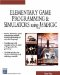Elementary Game Programming & Simulations Using Jamagic (Charles River Media Game Development)
ISBN: 1584502614
EAN: 2147483647
Year: 2002
Pages: 105
Authors: Sergio Perez